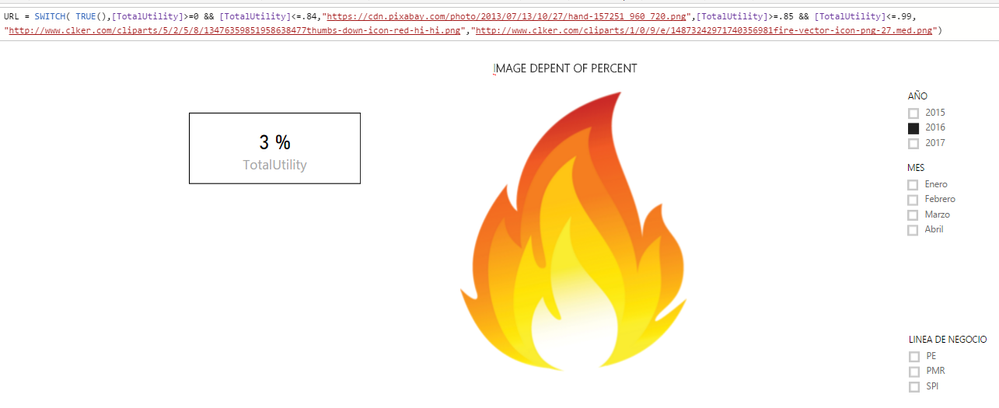cancel
Showing results for
Did you mean:Frequent Visitor

## IF three condition.

I have a measure in which I need depending on the percentage range, show an image that is on the web, so far only the one that showed me 1 image, does not fit into the other conditions.

I have a calculated field that brings me the utility percentage of the company.

The conditions are:

show image Green= 100% to infinity
show image Yellow= 85% to 99%
show image Red= 0% - 84%

For Example:

If the result of the operation  utility is: 75% will show the image yellow

I use this measure:

`Total_IMG = IF(AND([Totalutitlity %]>=0, [Totalutility%]<=.84),"https://cdn.pixabay.com/photo/2013/07/13/10/27/hand-157251_960_720.png",IF(AND([Totalutility %]>=.85,[Total pedidos %]<=.99),"http://www.clker.com/cliparts/5/2/5/8/13476359851958638477thumbs-down-icon-red-hi-hi.png","http://www.clker.com/cliparts/l/J/Q/d/1/X/yellow-thumbs-up-md.png")))`

The image loads on image display on img visualitation.

Thx, for ur help!!

1 ACCEPTED SOLUTIONMicrosoft

I am not sure what is your sample data, but you can check if the following steps return your expected result.

1. Create a new table using the following formula.

```image  =
UNION (
ROW ( "state", "Green",
"ImgURL", "https://cdn.pixabay.com/photo/2013/07/13/10/27/hand-157251_960_720.png" ),
ROW ( "state", "Red",
"ImgURL", "http://www.clker.com/cliparts/5/2/5/8/13476359851958638477thumbs-down-icon-red-hi-hi.png" ),
ROW ( "state", "yellow",
"ImgURL", "http://www.clker.com/cliparts/l/J/Q/d/1/X/yellow-thumbs-up-md.png" )
)```

2. Create a new measure using the following formula.

```LinkedMeasure  =
SUMX (
VALUES ( 'image '[state] ),
IF (
(
[Totalutility%]>= 0 && [Totalutility%] <= 0.84
&& VALUES ( 'image '[state] ) ="Green"
)
|| (
[Totalutility%] >= 0.85 && [Totalutility%] <= 0.99
&& VALUES ( 'image '[state] ) = "Red"
)
|| (
[Totalutility%] >=1
&& VALUES ( 'image '[state] ) = "yellow"
),
1
)
)```

3. Display image in ChicletSlicer. Image will change based on  the value of [Totalutility%] Measure.Thanks,
Lydia Zhang

Community Support Team _ Lydia Zhang
If this post helps, then please consider Accept it as the solution to help the other members find it more quickly.
11 REPLIES 11Community Champion

How about a SWITCH statement which is interannly converted into nested IFs?```Total_IMG =
SWITCH (
TRUE (),
[Totalutility %] >= 0
&& [Totalutility%] <= .84, "https://cdn.pixabay.com/photo/2013/07/13/10/27/hand-157251_960_720.png",
[Totalutility %] >= .85
&& [Total pedidos %] <= .99, "http://www.clker.com/cliparts/5/2/5/8/13476359851958638477thumbs-down-icon-red-hi-hi.png",
"http://www.clker.com/cliparts/l/J/Q/d/1/X/yellow-thumbs-up-md.png"
)```Frequent Visitor

Great solution, but still not working, I don't know why...Community Champion

Which part doesn't work?

Whats is the  [Totalutility %] Measure formula?Frequent Visitor

Totalutility is a measure... if i only left one condition, Even though it does not work, I think it is not considering the value of the result of measure Totalutility.. bacause if i change the number por example:

IMG = IF([Totalutility %]>=1,"https://cdn.pixabay.com/photo/2013/07/13/10/27/hand-157251_960_720.png","http://www.clker.com/clipar..."),

This example only shows green always, if change the value ">=1" for "<=1" shows a green image..Microsoft

I think your formula will only work if [TotalUtility %] is a column in your table rather than a calcualed measure.

Proud to be a Datanaut!Frequent Visitor

The problem is that I can not make the formula, as a calculated column because it is the division of the total of 2 different tablesMicrosoft

We can overcome that by creating a dynamic table that is based on two other tables.

It's a little more complex but can be done.  It would help to see your two tables so we can generate the formula you need.

Proud to be a Datanaut!Frequent Visitor

Thx for the help guys..

Any questions you have, please let me know. I am very grateful to take the time to help me, but I can not get this farFrequent Visitor

I finally found the way that the image works, now I can not make it work with 3 images ..

```Value = SUMX(
VALUES(Images[State]),
SWITCH(Images[State],
"ThumbsMedium",
IF([TotalUtility]>= .85,1,0),
IF([TotalUtility]>= .85,0,1)))```Microsoft

I am not sure what is your sample data, but you can check if the following steps return your expected result.

1. Create a new table using the following formula.

```image  =
UNION (
ROW ( "state", "Green",
"ImgURL", "https://cdn.pixabay.com/photo/2013/07/13/10/27/hand-157251_960_720.png" ),
ROW ( "state", "Red",
"ImgURL", "http://www.clker.com/cliparts/5/2/5/8/13476359851958638477thumbs-down-icon-red-hi-hi.png" ),
ROW ( "state", "yellow",
"ImgURL", "http://www.clker.com/cliparts/l/J/Q/d/1/X/yellow-thumbs-up-md.png" )
)```

2. Create a new measure using the following formula.

```LinkedMeasure  =
SUMX (
VALUES ( 'image '[state] ),
IF (
(
[Totalutility%]>= 0 && [Totalutility%] <= 0.84
&& VALUES ( 'image '[state] ) ="Green"
)
|| (
[Totalutility%] >= 0.85 && [Totalutility%] <= 0.99
&& VALUES ( 'image '[state] ) = "Red"
)
|| (
[Totalutility%] >=1
&& VALUES ( 'image '[state] ) = "yellow"
),
1
)
)```

3. Display image in ChicletSlicer. Image will change based on  the value of [Totalutility%] Measure.Thanks,
Lydia Zhang

Community Support Team _ Lydia Zhang
If this post helps, then please consider Accept it as the solution to help the other members find it more quickly.Frequent Visitor

Lydia Zhang,

I am very grateful for your response and above all for taking the time to help me, I have solved this issue.